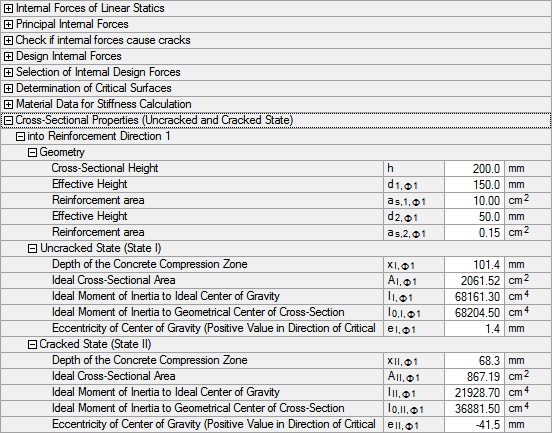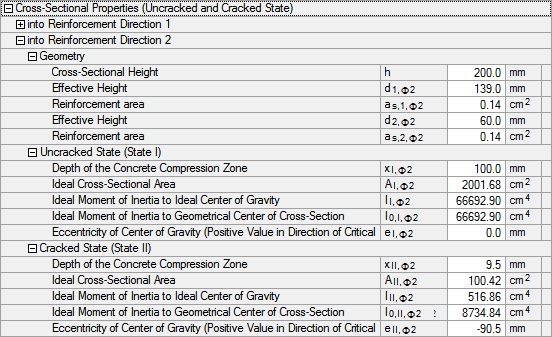# RF-CONCRETE Surfaces – Online Manual Version 5

Online manuals, introductory examples, tutorials, and other documentation.

# 2.7.10.5 Cross-section properties (cracked and uncracked state)

#### Cross-section properties (cracked and uncracked state)

The cross-section properties depend on the governing side and the reinforcement direction φ1. The minimum values are used for the reinforcement surfaces as2,φ1, as1,φ2, and as2,φ2.

The following cross-section properties for the uncracked and the cracked state are to be calculated in order to be able to assemble the stiffness matrix of the material D.

Centroid

The distance of the centroid of the ideal cross-section from the concrete surface in compression is calculated directly for the uncracked state.

For the cracked state, the depth χII,φ1 of the zone in compression must be calculated with the iterative method. Then, the distance of the centroid of the ideal cross-section from the surface in compression is calculated for the cracked state.

Ideal cross-section area Ac,d

The effective cross-section area in the uncracked state without the influence of creep is:

The effective cross-section area in the cracked state is determined with the influence of creep.

The coefficient α is the ratio of the moduli of elasticity of steel and concrete with or without the influence of creep.

Ideal moment of inertia to ideal centroid Ic,d

The effective moment of inertia to the ideal centroid in the uncracked state without the influence of creep is:

The effective moment of inertia to the ideal centroid in the cracked state is determined with the influence of creep.

Ideal moment of inertia to the geometric center of the cross-section I0,c,d

The ideal moment of inertia to the geometric center of the cross-section in the uncracked state without the influence of creep is:

The ideal moment of inertia to the geometric center of the cross-section in the cracked state is determined with the influence of creep.

Eccentricity of centroid ec,d

The eccentricity of the ideal centroid of the cross-section is determined as follows:

• uncracked state:

• cracked state:Figure 2.120 Cross-sectional properties in reinforcement direction 1Figure 2.121 Cross-sectional properties in reinforcement direction 2